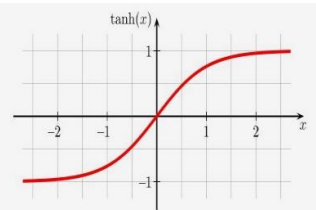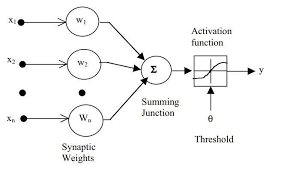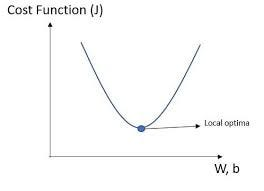# Neural network architecture

Neural network is made of layers with many interconnected nodes (neurons).There are three main layers, specifically input layer, hidden layer and output layer. There can be more than one hidden Layer. As the hidden layers increase the complexity of the neural network also increases.

# Activation function

Activation function is a mechanism by which the artificial neuron processes incoming information and passes it throughout the network. Threshold activation function is called so as it results in an output signal only once a specified input threshold has been attained. Different types of activation functions are unit step activation function, sigmoid activation function, hyperbolic tangent activation function and rectified linear unit activation function.

## c. Hyperbolic Tangent Activation Function

This function is also known as tanh, a transfer function. It is widely used in deep neural networks .Hyperbolic tangent activation function particularly serve as the activation function in recurrent neural networks. This is a shifted version of sigmoid activation. The output of this function has a wider range of values.Graph depicting hyperbolic tangent activation function

# Synaptic weights

The synaptic weights of neurons are determined based on the neural net learning process (Learning Algorithm) . Most common measure of the error (cost function) is mean square error, E = (y — d)² . Iterations of the above process of providing the network with an input and updating the network’s weight to reduce error is used to train the network.Figure showing the synaptic weights in a neural network

# Cost function

Cost Function is a loss function, a function to be minimized . In ANN, the cost function is used to return a number to indicate how well the neural network performed to map training examples to correct output.Graph depicting the cost function variation

# Learning rate

Learning rate is a hyperparameter that controls how much to change the model in response to the estimated error each time the model weights are updated. Choosing the learning rate is challenging. A small learning rate value may result in a long training process that could get stuck while a large value may result in learning a sub-optimal set of weights too fast or an unstable training process.